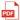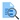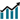# Interactive Multimedia in Learning 2- Dimensional Gemoetric Shapes in Elementary School

• Jesi Alexander Alim
• Edwin Musdi

### Abstract

Geometry is a branch of mathematics
that occupies a special position in learning mathematics
in schools. The importance of the concepts contained
therein places geometry in relatively large proportions
in the curriculum. A two-dimensional geometric shape
is a flat plane shape that has two dimensions. It is a flat
area that is bounded by straight or curved lines.
Geometry has several different calculation formulas
and some of the two-dimensional geometric shapes are
known as square, rectangle, triangle, trapezoid,
parallelogram, kite, rhombus and circle. The problem is
how to apply a learning method or media that is
enjoyable supporting by an interesting visualization.
The choice can be an application that can be done with
interactive learning multimedia so that students can
easily understand geometry and fosters tudents’
interest in learning mathematics.

Published
2019-12-09
Section
EducationpdfAbstract views: 8downloads: 9
s